# Learn Embedded Programming [Step by Step]

## Embedded Programming Tutorials for Beginners with Step By Step Guide.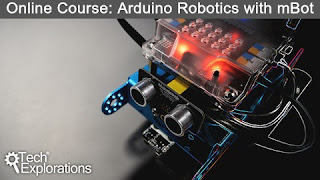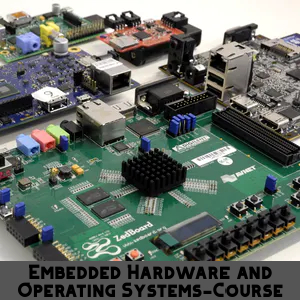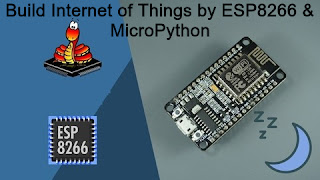## Soil Moisture Meter Project Using Microcontroller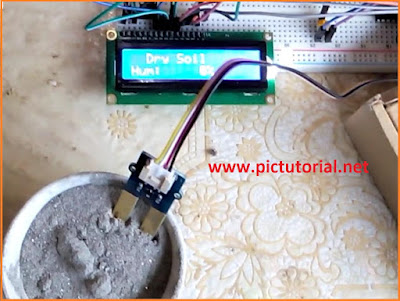Microcontroller Based Soil Moisture Meter

## Grove - Moisture Sensor :

Basically grove moisture sensor is used to measuring the moisture of the soil. It also can be used as water sensor. We can water  plants according to its humidity by using the sensor .  Look at the sensor pictures and its pin out.Soil Moisture Meter Project Using Microcontroller

## Basic Concepts:

The Moisture sensor will be connected with a pic microcntroller and a LCD display will also be connected with microcontroller . Moisture sensor will provide voltage into microcontroller's RA1 pin(ADC) according to the humidity of soil . It will provide reading from (0-721). After calculating the percentage and soil condition , the microcontroller sends out that data to the LCD Display . That's the basic concept .

## ADC(Analog to Digital Converter ) :

We need a basic knowledge about ADC . Let's  take a look at here :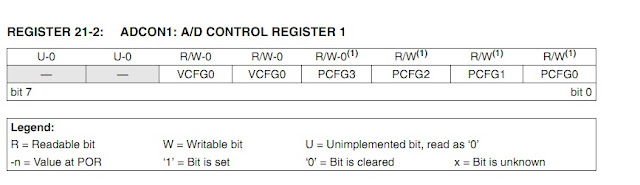ADCON1 Register

Basically ADC is like as voltage divider . According to voltage It produce output
bit 5 : VCFG0: Voltage Reference Configuration bit (VREF- source)
1 = VREF- (AN2)
0 = VSS or 0 volt

bit 4 :VCFG0: Voltage Reference Configuration bit (VREF+ source)
1 = VREF+ (AN3)
0 = VDD or 5volt

We will set  VCFG0[bit 5]=0and VCFG0[bit4]=0 . So we will get highest value 5volt and lowest value 0volt.The ADCON1 is a 10 bit register that means  2 to the power 10  is it's highest counting capacity and result is 1024 . So this register can count from 0 to 1023 . When 0 volt , we get reading at RA1 pin  0 .When 5 volt , we get reading at RA1 pin 1023. It means 5volt equivalent to 1023 .

As we know in water the sensor provide 722 data and  absolutely it should be 100% .
So     ""        1     ""      (100/744) %

## MikroC Source Code :

``````

sbit LCD_RS at LATB7_bit;
sbit LCD_EN at LATB6_bit;
sbit LCD_D4 at LATB5_bit;
sbit LCD_D5 at LATB4_bit;
sbit LCD_D6 at LATB3_bit;
sbit LCD_D7 at LATB2_bit;
sbit LCD_RS_Direction at TRISB7_bit;
sbit LCD_EN_Direction at TRISB6_bit;
sbit LCD_D4_Direction at TRISB5_bit;
sbit LCD_D5_Direction at TRISB4_bit;
sbit LCD_D6_Direction at TRISB3_bit;
sbit LCD_D7_Direction at TRISB2_bit;
// End LCD module connections
double source=0;
int view=0;
char txt;
void main() {
ADCON1=0x0D;         // Configure RE1 pin as input
CMCON=7;
TRISC.F4=0;
Lcd_Init();            // Initialize LCD
Lcd_Cmd(_LCD_CLEAR);        // Clear display
Lcd_Cmd(_LCD_CURSOR_OFF);     // Cursor off

while(1){
source=100*source;
source=source/744;
inttostr(source,txt);
if(source>96){
source=100;
}
if(source>83){
Lcd_Cmd(_LCD_CLEAR);
Lcd_Out(1,3, "Water!!");
Lcd_Out(2,1, "Hum:");
Lcd_Out(2,5,txt);
Lcd_Chr(2,11,0x25);
Lcd_Chr(2,12,' ');
Lcd_Chr(2,13,' ');
Lcd_Chr(2,14,' ');
Lcd_Chr(2,15,' ');
Lcd_Chr(2,16,' ');
delay_ms(1000);
}
else if(source>40&&source<=83){
Lcd_Cmd(_LCD_CLEAR);
Lcd_Out(1,3, "Humid Soil");
Lcd_Out(2,1, "Hum:");
Lcd_Out(2,5,txt);
Lcd_Chr(2,11,0x25);
Lcd_Chr(2,12,' ');
Lcd_Chr(2,13,' ');
Lcd_Chr(2,14,' ');
Lcd_Chr(2,15,' ');
Lcd_Chr(2,16,' ');
PORTC.f4=0; //motor off
delay_ms(1000);
} else{
Lcd_Cmd(_LCD_CLEAR);
Lcd_Out(1,3, "Dry Soil");
Lcd_Out(2,1, "Hum:");
Lcd_Out(2,5,txt);
Lcd_Chr(2,11,0x25);
Lcd_Chr(2,12,' ');
Lcd_Chr(2,13,' ');
Lcd_Chr(2,14,' ');
Lcd_Chr(2,15,' ');
Lcd_Chr(2,16,' ');
PORTC.f4=1;  // motor on
delay_ms(1000);
PORTC.f4=1;
}
}
}

``````

## Circuit :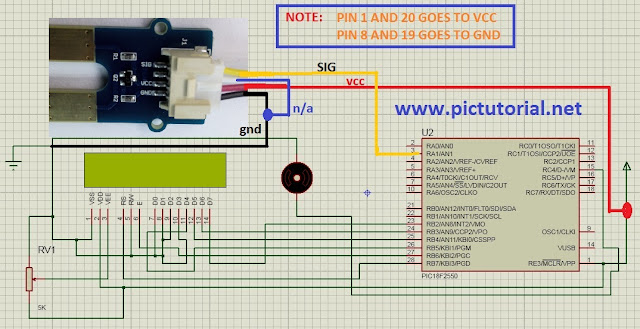Microcontroller Based Soil Moisture Meter circuit

## Result:Soil Moisture Meter Project Using Microcontroller

## Gas Sensor (MQ-9) :

MQ-9 Gas Sensor is one kind of semiconductor which has very lower conductivity in open air. But in Carbon Monoxide, Methane and LPG gas it has good conductivity. By using ADC of pic microcontroller we can measure it. Let's take a look on MQ-9 sensor .

## Basic Concepts :

The MQ-9 sensor will be connected with a pic microcntroller and a buzzer will also be connected with microcontroller. When LPG gas will be detected in sensor, it will provide voltage into microcontroller's RA1 pin(ADC). It will provide reading more than 590. At this situation microcontroller will turn on the buzzer and LED. That's the basic concept.

## ADC(Analog to Digital Converter ) :

We need a basic knowledge about ADC. Let's  take a look at here :ADCON1 Register

Basically ADC works like as a voltage divider. According to voltage It produce output.

bit 5 : VCFG0: Voltage Reference Configuration bit (VREF- source)
1 = VREF- (AN2)
0 = VSS or 0 volt

bit 4 :VCFG0: Voltage Reference Configuration bit (VREF+ source)
1 = VREF+ (AN3)
0 = VDD or 5volt

We will set  VCFG0[bit 5]=0and VCFG0[bit4]=0. So we will get highest value 5volt and lowest value 0volt.The ADCON1 is a 10 bit register that means  2 to the power 10  is it's highest counting capacity and result is 1024. So this register can count from 0 to 1023. When 0 volt, we get reading at RA1 pin  0. When 5 volt, we get reading at RA1 pin 1023. It means 5volt equivalent to 1023 .

If  5 volt    equal    reading 1023 .

So 1  volt   equal    reading 1023/5
[When LPG Gas detect MQ-9 Sensor provide more than 2.93 volt at AO Pin]
So 3 volt equal  reading  (1023/5)*2.93 =599 When we get reading 599 at ADC channel, we understand  that sensor detects LPG. So it makes PORTB.F6 pin high and Buzzer turns on .

## MikroC Source Code :

``````

double source=0;
char txt;
void main() {
ADCON1=0x0D;         // Configure RA1 pin as input
CMCON=7;
TRISB.F6=0;

while(1){
PORTB.F6=0;
if(source>600){
delay_ms(500);
PORTB.F6=1;
}
}
}
``````

## Thank You!

Ain't getting any visitors!

## Featured Post

### Rs 232 , Serial Communication with PIC Microcontroller in Proteus [step by step details]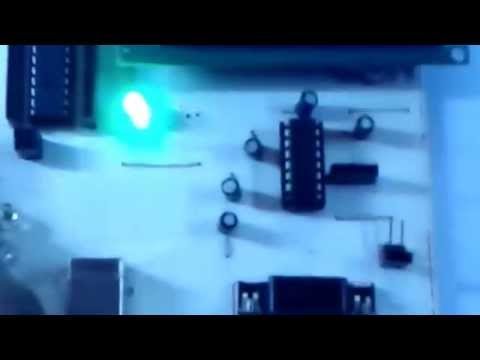## Tags

: (1) 18F2550 (1) 36KHz (3) and (1) arduino (1) Based (1) battery (1) Bipolar (1) Blinking (1) blinks (1) Bluetooth (1) button (1) circuit (1) clock (1) control (1) Db9 (1) DC Motor (2) digital (2) display (2) DS1307 (1) electronic (1) flash (1) flashing (1) HC-06 (1) home (1) how (1) How to (10) i2c tutorial (1) in (1) indicator (1) interface (8) interfacing (3) Interrupt (3) Introduction (1) IR Receiver (4) key pad (1) keyboard (1) keypad (1) lavel (1) Lcd 16x2 (2) lcd 2x16 (2) led (1) lm35 (2) LPG (1) machine (1) make (1) making (1) matrix (1) max232 (1) meter (2) microchip (4) microchips (3) mikroC (5) musical (1) NEC Protocol (4) pcb (5) PIC (3) pic controller (11) pic proteus (1) Pic Tutorial (12) pic18 (2) pic18f2550 (11) picRFモジュール (1) proteus (6) push (1) push button (1) PWM (1) real (1) Rs 232 (1) Rs232 (1) scroll (1) scrolling (1) Serial Port (1) simulation (2) step by step (7) step bystep (1) text (2) time (1) timer (4) timer0 (4) tone (1) tutorial (2) Unipolar (1) USB (1) usb 1.0 (1) USB HID (1) using (9) voltmeter (1) voting (1) with (2) work (1)

## Traffic Feed

Live Traffic Feed
Visitor Tracking